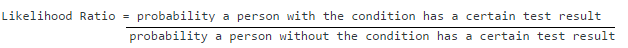# Likelihood Ratio (Medicine): Basic Definition, Interpretation

Share on

Statistics Definitions > Likelihood Ratio

The following article covers the Likelihood Ratio as it applies to diagnostic tests in medicine. If you are looking for the test used to choose a best model, see the next article: Likelihood Ratio Test (Probability and Mathematical Statistics).

## What is a Likelihood Ratio?

Likelihood ratios (LR) in medical testing are used to interpret diagnostic tests. Basically, the LR tells you how likely a patient has a disease or condition. The higher the ratio, the more likely they have the disease or condition. Conversely, a low ratio means that they very likely do not. Therefore, these ratios can help a physician rule in or rule out a disease.

## Formulas

The formula for the likelihood ratio (LR) is:Tests can be either positive or negative, so there are two ratios:

• Positive LR: This tells you how much to increase the probability of having a disease, given a positive test result. The ratio is:
Probability a person with the condition tests positive (a true positive) /
probability a person without the condition tests positive (a false positive).
• Negative LR: This tells you how much to decrease the probability of having a disease, given a negative test result. The ratio is:
Probability a person with the condition tests negative (a false negative) /
probability a person without the condition tests negative (a true negative).

Sensitivity and specificity are an alternative way to define the likelihood ratio:

• Positive LR = sensitivity / (100 – specificity).
• Negative LR = (100 – sensitivity) / specificity.

## Interpreting Likelihood Ratios

Likelihood ratios range from zero to infinity. The higher the value, the more likely the patient has the condition. As an example, let’s say a positive test result has an LR of 9.2. This result is 9.2 times more likely to happen in a patient with the condition than it would in a patient without the condition.

A rule of thumb (McGee, 2002; Sloane, 2008) for interpreting them:

• 0 to 1: decreased evidence for disease. Values closer to zero have a higher decrease in probability of disease. For example, a LR of 0.1 decreases probability by -45%, while a value of -0.5 decreases probability by -15%.
• 1: no diagnostic value.
• Above 1: increased evidence for disease. The farther away from 1, the more chance of disease. For example, a LR of 2 increases the probability by 15%, while a LR of 10 increases the probability by 45%. An LR over 10 is very strong evidence to rule in a disease.

## Real Life Example

Sloane (2008) offers the following example for a serum ferritin test, which test for iron deficiency anemia. The LR for the test is:

 Result (mg/dl) Likelihood Ratio ≤ 15 51.8 15 – 24 8.8 25 – 34 2.5 45 – 100 0.5 ≥ 100 0.08

The LR of 51.8 for the under 15 mg/dL result very strong evidence to rule in iron deficiency anemia. On the other hand, the very low LR of 0.08 is clear evidence there is no anemia. Scores in between are open to interpretation; further tests may be needed.

## Bayes Theorem and the LR

In theory, the LR tells you if a test is correct. In practice, it isn’t used very much. This could be because Bayes’ Theorem (the theory behind pre-test and post-test probabilities) is not very easy to understand. However, you don’t need to comprehend the inner workings of the theorem to understand the Likelihood ratio form of the theorem:

Post-Test Odds = Pre-test Odds * LR.

For example, let’s say a patient returning from a vacation to Rio presents with a fever and joint pain. Past data tells you that 70% of patients in your practice who return from Rio with a fever and joint pain have Zika. The blood test result is positive, with a likelihood ratio of 6. To calculate the probability the patient has Zika:
Step 1: Convert the pre-test probability to odds:
0.7 / (1 – 0.7) = 2.33.
Step 2: Use the formula to convert pre-test to post-test odds:
Post-Test Odds = Pre-test Odds * LR = 2.33 * 6 = 13.98.
Step 3: Convert the odds in Step 2 back to probability:
(13.98) / (1 + 13.98) = 0.93.
There is a 93% chance the patient has Zika.

Reference:
McGee, S. Simplifying Likelihood Ratios. J Gen Intern Med. 2002 Aug; 17(8): 647–650. Available here.
Sloane, P. 2008. Essentials of Family Medicine. Lippincott Williams & Wilkins.

CITE THIS AS:
Stephanie Glen. "Likelihood Ratio (Medicine): Basic Definition, Interpretation" From StatisticsHowTo.com: Elementary Statistics for the rest of us! https://www.statisticshowto.com/likelihood-ratio/
---------------------------------------------------------------------------Need help with a homework or test question? With Chegg Study, you can get step-by-step solutions to your questions from an expert in the field. Your first 30 minutes with a Chegg tutor is free!